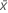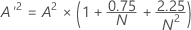# Methods and formulas for Normality Test

Select the method or formula of your choice.

## Mean

A commonly used measure of the center of a batch of numbers. The mean is also called the average. It is the sum of all observations divided by the number of (nonmissing) observations.

### Notation

TermDescription
xiith observation
Nnumber of nonmissing observations

## Standard deviation (StDev)

The sample standard deviation provides a measure of the spread of your data. It is equal to the square root of the sample variance.

### Formula

If the column contains x 1, x 2,..., x N, with mean, then the standard deviation of the sample is:

### Notation

TermDescription
x i i th observationmean of the observations
N number of nonmissing observations

## N

Minitab displays the number of nonmissing observations in a sample.

## Anderson-Darling statistic (A2)

A2 measures the area between the fitted line (which is based on the chosen distribution) and the nonparametric step function (which is based on the plot points). The statistic is a squared distance that is weighted more heavily in the tails of the distribution. A small Anderson-Darling value indicates that the distribution fits the data better.

The Anderson-Darling normality test is defined as:

H0: The data follow a normal distribution

H1: The data do not follow a normal distribution

### Notation

TermDescription
F(Yi), which is the cumulative distribution function of the standard normal distribution
Yiordered data

## Ryan-Joiner

The Ryan-Joiner test provides a correlation coefficient, which indicates the correlation between your data and the normal scores of your data. If the correlation coefficient is near 1, your data falls close to the normal probability plot. If it is less than the appropriate critical value, you will reject the null hypothesis of normality.

### Formula

The correlation coefficient is calculated as:

### Notation

TermDescription
Yi ordered observations
bi normal scores of your ordered data
s2 sample variance

## Kolmogorov-Smirnov

### Formula

The Kolmogorov-Smirnov test is defined as:
• H0: The data follow a normal distribution
• H1: The data do not follow a normal distribution
The Kolmogorov-Smirnov test statistic is defined as:

### Notation

TermDescription
D+ maxi {i / nZ (i)}
D maxi {Z (i) – (i – 1) / n)}
Z F(X(i))
F(x)probability distribution function of the normal distribution
X(i) ith order statistics of a random sample, 1 ≤ i ≤ n
n sample size

## P-value

Another quantitative measure for reporting the result of the normality test is the p-value. A small p-value is an indication that the null hypothesis is false.

If you know A2 you can calculate the p-value. Let:
Depending on A'2, you will calculate p with the following equations:
• If 13 > A'2 > 0.600 then p = exp(1.2937 - 5.709 * A'2 + 0.0186(A'2)2)
• If 0.600 > A'2 > 0.340 then p = exp(0.9177 - 4.279 * A'2 – 1.38(A'2)2)
• If 0.340 > A'2 > 0.200 then p = 1 – exp(–8.318 + 42.796 * A'2 – 59.938(A'2)2)
• If A'2 <0.200 then p = 1 – exp(–13.436 + 101.14 * A'2 – 223.73(A'2)2)

## Plot points

In general, the closer the points fall to the fitted line, the better the fit. Minitab provides two goodness-of-fit measures to help assess how the distribution fits your data.

### Formula

The table below shows how the middle line is constructed:
Distribution x coordinate y coordinate
Normal x Φ–1 norm

### Notation

TermDescription
Φ–1 norm value returned for p by the inverse cdf for the standard normal distribution

## Probability plots

The input data are plotted as the x-values. Minitab calculates the probability of occurrence without assuming a distribution. The Y-scale on the graph resembles the Y scale found on normal probability paper where the probabilities plot as a straight line, as if the data are from a normal distribution.

By using this site you agree to the use of cookies for analytics and personalized content.  Read our policy